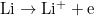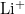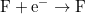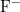×
Get Full Access to Introductory Chemistry - 5 Edition - Chapter 4 - Problem 82p
Get Full Access to Introductory Chemistry - 5 Edition - Chapter 4 - Problem 82p

×

# Determine the number of protons and electrons in each ion.(a) Al3+(b) S2?(c) I?(d) Ag+ISBN: 9780321910295 34

## Solution for problem 82P Chapter 4

Introductory Chemistry | 5th Edition

• Textbook Solutions
• 2901 Step-by-step solutions solved by professors and subject experts
• Get 24/7 help from StudySoup virtual teaching assistantsIntroductory Chemistry | 5th Edition

4 5 1 300 Reviews
29
4
Problem 82P

Determine the number of protons and electrons in each ion.

(a)  $$\mathrm{Al}^{3+}$$

(b)  $$\mathrm{S}^{2-}$$

(c)  $$\mathrm{I}^{-}$$

(d)  $$\mathrm{Ag}^{+}$$

Step-by-Step Solution:

Step 1 of 5

Here, we are going to calculate the number of protons and electrons of each ion.

Mass Number: The sum of the number of protons and neutrons in an atom is called its mass number. This is denoted by A.

Atomic number: The number of protons present in the nucleus in an atom is called its atomic number. It is denoted by Z.

We know,

The number of protons and electrons are always same for a neutral atom. Thus, ions are formed by losing or gaining of electrons. A positively charged ion is formed when an atom loses its electron. So no. of protons always remain same. For example:Here, no of protons inion will be = 3

But the electrons will be = 3 - 1 = 2 e

Similarly,

A negatively charged ion is formed when atoms gain electrons.

For example :Total no of protons ofion will be same as neutral F,

But the no electrons ofion will be = 9 + 1= 10

Step 2 of 5

Step 3 of 5

##### ISBN: 9780321910295

This full solution covers the following key subjects: determine, electrons, ion, Protons. This expansive textbook survival guide covers 19 chapters, and 2046 solutions. The full step-by-step solution to problem: 82P from chapter: 4 was answered by , our top Chemistry solution expert on 05/06/17, 06:45PM. This textbook survival guide was created for the textbook: Introductory Chemistry, edition: 5. Introductory Chemistry was written by and is associated to the ISBN: 9780321910295. The answer to “?Determine the number of protons and electrons in each ion.(a) $$\mathrm{Al}^{3+}$$(b) $$\mathrm{S}^{2-}$$(c) $$\mathrm{I}^{-}$$(d) $$\mathrm{Ag}^{+}$$” is broken down into a number of easy to follow steps, and 14 words. Since the solution to 82P from 4 chapter was answered, more than 1460 students have viewed the full step-by-step answer.

## Discover and learn what students are asking

Calculus: Early Transcendental Functions : Second-Order Nonhomogeneous Linear Equations
?Finding a Particular Solution In Exercises 5-10,find a particular solution of the differential equation. y’’ - 2y’ - 15y = sin x

Statistics: Informed Decisions Using Data : Properties of the Normal Distribution
?In Problems 25–28, the graph of a normal curve is given. Use the graph to identify the values of ? and ?.

Unlock Textbook Solution